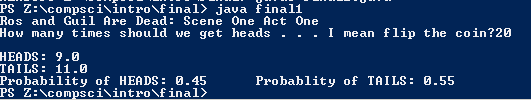# First Semester Final

```//Name: Ilana Levy
//Program Name: First Semester Final
//File Name: final1.java
//Date: 1/20/2016

import java.util.Random;
import java.util.Scanner;

public class final1
{
public static void main( String[] args)
{
Random r = new Random();
Scanner keyboard = new Scanner(System.in);

int numFlip;
double numHeads = 0, numTails = 0, probHead = 0, probTails = 0;

System.out.print( "Ros and Guil Are Dead: Scene One Act One\nHow many times should we get heads . . . I mean flip the coin?");

///Using do-while to make sure that number of coin flips is more than 0
do
{
numFlip = keyboard.nextInt();
if (numFlip < 1)
{
System.out.print( "Nice Try. Now A real number");
}
}
while ( numFlip < 1);

///for loop for to flip the coin the correct number of times
for ( int i = 0; i < numFlip; i++)
{
int x = r.nextInt(2);
if ( x == 1)
{

}
else if (x == 0)
{

numTails = numTails+ 1;
///if tails, number of overall tails counted goes up
}
else
{
System.out.println( "Error");
}
}

System.out.println( "Probability of HEADS: " + probHead + "\tProbablity of TAILS: " + probTails);
/*It is impossible to consistently get 50% with a finite number that is not infinity. As the number
of flips increases, the probability gets closer and closer to 50%. Thereford, in this case where we
use an integer for the number  of flips, the max number of flips is 2,100,000,000 which will give us
the closest probability consistently to 50%.
*/

/*Ros and Guil Probability Quotes:
A weaker man might be moved to re-examine his faith, if in nothing else at least in the law of probability

It must be indicative of something, besides the redistribution of wealth. (He muses.) List of possible
explanations.
One: I'm willing it. Inside where nothing shows, I'm the essence of a man spinning double-headed
coins, and betting against himself in private atonement for an unremembered past.
Two: time has stopped dead, and a single experience of one coin being spun once has been repeated ninety times... (He flips a coin, looks at it, tosses it to ROS.) On the whole, doubtful.
Three: divine intervention,that is to say, a good turn from above concerning him, cf. children of Israel, or retribution from above concerning me, cf. Lot's wife.
Four: a spectacular vindication of the principle that each individual coin spun individually (he spins one) is as likely to come down heads as tails and therefore should cause no surprise that each individual
time it does. (It does. He tosses it to ROS.)

*/
}
}
```